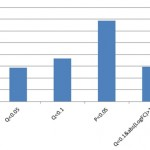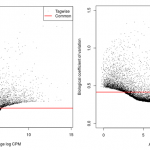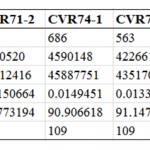# Some key factors for number of significant DE genes

In the different RNA-Seq experiments, we have found that some experiments have more significant DE (differential expression) genes than others. Besides the experiment design which is the most important factor, a number of other factors may influence the number of DE genes. Here, we list the different experiments that we have carried out the investigation of the factors that may influence the number of DE genes.

Previous analyses applying gentle trimming have negligible effects on the number of DE genes. However, heavy trimming could decrease the expression level heavily which is not objective for analysis.

The threshold of Significant DE genes

The default parameter forchoosing the significance of a DE gene is the Q-value (i.e. FDR P-value)< 0.05. This is based on the Benjamini–Hochberg procedure correction. One way to change the number of DE genes is to change the threshold value or the parameter used as a threshold. For example, any of Q-value< 0.1, P-value<0.05 and Q-value<0.1&abs(Log2FC)>1 could be used as threshold parameters. Using data sequenced at the CVR (treated and untreated cells) as an example, edgeR is used as the DE analysis tool (Fig. 1). The largest number of deferentially expressed genes is found if P<0.05 is used as a threshold parameter.On the other hand, if you want to reduce the number of DE genes, you could change the correction procedure used. For example, the Benjamini–Yekutieli procedure is more conservative than the Benjamini–Hochberg procedure.Fig.1 Number of significant DE genes with different threshold

Biological coefficient of variation

An important factor that influences the number of DE genes is the variation among the samples.The BCV (Biological Coefficient of Variation) plot is a way to measure the biological variation within a particular condition. A common dispersion (i.e. red line on the BCV plot) between 0.2 and 0.4 is usually considered reasonable and hence could detect more DE genes.If the common dispersion is above the 0.4 threshold, this will influence the number of DE genes found in the study. Additionally, the PCA (principal component analysis) and MDS (multidimensional scaling) plots which represents the relationship between groups of samples are affected by high BCVs. The experiment was run twice. We can see the differences in the BCV and MDS plots between the two experiments. An experiment where the common dispersion is 0.41 provides almost 576 DE genes whilst an experiment with high average BCV results in only 109 DE genes. Additionally, the MDS plots clearly differentiate the different conditions when the common dispersion is low implying greater variation between the conditions than within, as expected.

experiment 1 (Condition 1; Condition 2):

Condition 1 sample ID: CVR71-1, CVR74-1, CVR57-1;

Condition 2 sample ID: CVR71-2, CVR74-2, CVR57-2

experiment 2 (Condition1; Condition 2):

Condition 1 sample ID: CVR82-1, CVR 82-2, CVR82-3;

Condition 2 sample ID: CVR83-1, CVR83-2, CVR83-3Fig 2. BCV plot of Experiment 1 vs BCV plot of Experiment 2Fig 3. MDS plot of Experiment 1 vs MDS plot of Experiment 2

Number of samples

Statistically, it is beneficial to have as many replicates as possible. The minimum number of replicates would be 3 but it is advisable to have more than 4 in each condition for cell lines and 6 for tissue samples that inherently have more variation in the genes being expressed. The number of DE genes would be affected by number of samples in the experiment. We used edgeR to compare the results obtained with 2 replicates versus 3 replicates for an experiment 1 and cell experiment 2 by edgeR and get the result to Fig. 4. Figure 4 shows that we could get more significantly DE genes with more samples.Fig.4 Number of significant DE genes vs sample number

However, if we have outlier samples in each condition, the number of significant DE genes will decrease (Fig. 5). For example, we made a new DE analysis of the pig genome using:

Condition 1:CVR57-1, CVR82-1, CVR 82-2, CVR82-3

Condition 2: CVR57-2, CVR83-1, CVR83-2, CVR83-3

As shown in the Fig.5, the number of DE genes decreased because of the outlier (i.e. CVR57-1 and CVR57-2). By adding this outlier, you are increasing the biological variation in the experiment, resulting in a higher BCV and a lower number of DE genes.

Number of reads in each sample

The number of DE genes could also be affected by the number of reads in the samples. Here we randomly sub-sampling the number of RNA-Seq reads of each sample (Fig. 5). If we have more reads, we find more DE genes.DESeq2 is influenced more by the number of reads in the experiment than edgeR which is less affected by changes in the number of mapped reads. This also suggests that sequencing 25% less per sample, will not adversely affect the number of DE genes determined by edgeR.Fig.5 Number of significant DE genes vs proportion of sub-sampling

Contamination

Here we test some of our samples (unmapped reads) to the Mycoplasmas bacteria with Ion-Proton sequencing data. Mycoplasma can influence the growth of cell cultures may adversely affect the experiment, thus resulting in lower numbers of DE genes.

The mycoplasma genomes used in this study were downloaded from NCBI genomes: Mycoplasma hominis ATCC 23114 (NC_013511.1), M. hyorhinis MCLD (NC_017519.1), Mycoplasma fermentans M64 (NC_014921.1) and Acholeplasma laidlawii PG-8A (NC_010163.1). As shown in the Table 1, there is not an obvious relationship between number of significant DE genes and the mycoplasma contamination.

Table 1 Statistic of mapping to Mycoplasma and pig genomeThe number of genes in the genome

The number of genes in the genome could also influence the number of significant DE genes although this is unlikely to be the case in the current set of experiments approx. 25,000 genes and approx. 26,000.

The statistical model

Even in the same pipeline, use different statistical model could affect the number of DE genes as well.

For example, number of DE genes will increase from 576 to 3457 if the model of edgeR changed from ‘classical linear models’ to ‘Generalized linear models (GLM) with muti-factor of samples’ .

Summary

We propose that the following strategy for future experimental design:

• increasing the number of replicates;
• the number of sequenced reads could be decreased slightly to accommodate for more replicates;
• insuring consistent handling in the laboratory.